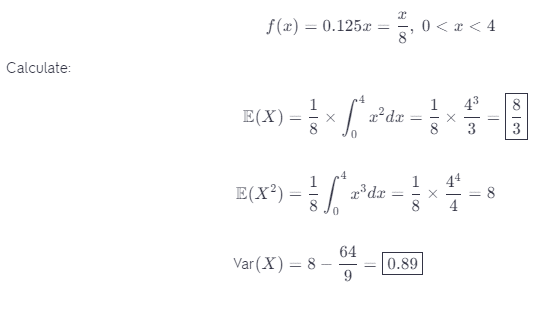# Suppose F(X) = 0.125x For 0 < X < 4. Determine The Mean And Variance Of X.

We thoroughly check each answer to a question to provide you with the most correct answers. Found a mistake? Let us know about it through the REPORT button at the bottom of the page.

Suppose that f(x) = 0.125x for 0 < x < 4. Determine the mean and variance of X.